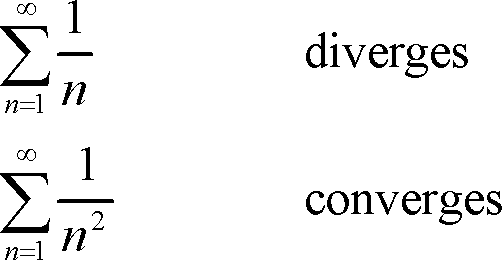# Series Convergence & Estimation Assessment Test

10 Questions | Total Attempts: 104SettingsTake our intelligent assessment test to evaluate your knowledge of how series converges and diverges, and the value a series converges to — called series convergence and estimation.

• 1.
A series can be defined as...
• A.

• B.

Collection of numbers

• C.

Line of figures

• D.

Sequence of sums

• 2.
A series that converges has a...
• A.

Finite limit

• B.

Function

• C.

Slope

• D.

Integral

• 3.
A series that diverges means the partial sums have no...
• A.

Standard

• B.

Function

• C.

Approach infinity

• D.

Line

• 4.
A series that converges has a number that is...
• A.

Approached

• B.

Fine

• C.

Done

• D.

Over

• 5.
A sequence which does not converge is said to be...
• A.

Open

• B.

Divergent

• C.

Over

• D.

Broken

• 6.
The fundamental notion on which the whole of analysis ultimately rests is the...
• A.

Function of a sequence

• B.

Nomination

• C.

Limit of a sequence

• D.

Or donation

• 7.
The set of values for which a series converges is the...
• A.

Positioning

• B.

Power rule

• C.

Limiting power

• D.

Interval of convergence

• 8.
A fundamental concept in calculus and analysis concerning the behavior of that function near a particular input is...
• A.

Power

• B.

Limit of a function

• C.

Theorem

• D.

Input theory

• 9.
A limit converges if it has a...
• A.

Finite value

• B.

Number

• C.

Figure

• D.

Value

• 10.
An interval in which a power series converges is called the...
• A.

• B.

Limit line

• C.

Number line

• D.

Limitation

Related TopicsBack to top